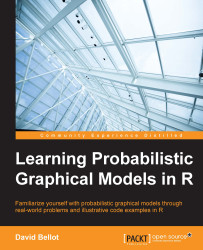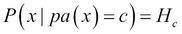•#### Learning Probabilistic Graphical Models in R#### Overview of this book

Probabilistic graphical models (PGM, also known as graphical models) are a marriage between probability theory and graph theory. Generally, PGMs use a graph-based representation. Two branches of graphical representations of distributions are commonly used, namely Bayesian networks and Markov networks. R has many packages to implement graphical models. We’ll start by showing you how to transform a classical statistical model into a modern PGM and then look at how to do exact inference in graphical models. Proceeding, we’ll introduce you to many modern R packages that will help you to perform inference on the models. We will then run a Bayesian linear regression and you’ll see the advantage of going probabilistic when you want to do prediction. Next, you’ll master using R packages and implementing its techniques. Finally, you’ll be presented with machine learning applications that have a direct impact in many fields. Here, we’ll cover clustering and the discovery of hidden information in big data, as well as two important methods, PCA and ICA, to reduce the size of big problems.
Learning Probabilistic Graphical Models in RCreditswww.PacktPub.comPrefaceFree Chapter
Probabilistic ReasoningExact InferenceLearning ParametersBayesian Modeling – Basic ModelsApproximate InferenceBayesian Modeling – Linear ModelsProbabilistic Mixture ModelsAppendixIndex## Maximum likelihood

This section introduces a simple algorithm to learn all the parameters of a graphical model as we saw until now. In the first section, we had our first experience of learning such a model and we concluded by saying that the parameters can be learned locally for each variable. It means that, for each variable x having parents pa(x) in the graph, for each combination of the parents pa(x) we compute frequencies for each value of x. If the dataset is complete enough, then this leads to the maximum likelihood estimation of the graphical models.

For each variable x in the graphical modeling, and for each combination c of the values of the parents of pa(x) of x:

• Extract all the data points corresponding to the values in c

• Compute a histogram Hc on the value of x

• AssignIs that it? Yes it is, it's all you have to do. The difficult part is the extraction of the data points, which is a problem you can solve in R using the `ddply` or `aggregate` functions.

But why is it so simple? Before...